# Histogram bins and binwidth in ggplot2

## Default histogram

By default, the underlying computation of `geom_histogram` through `stat_bin` uses 30 bins, which is not always a good default.

``````# install.packages("ggplot2")
library(ggplot2)

# Data
set.seed(05022021)
x <- rnorm(600)
df <- data.frame(x)

# Default histogram
ggplot(df, aes(x = x)) +
geom_histogram()``````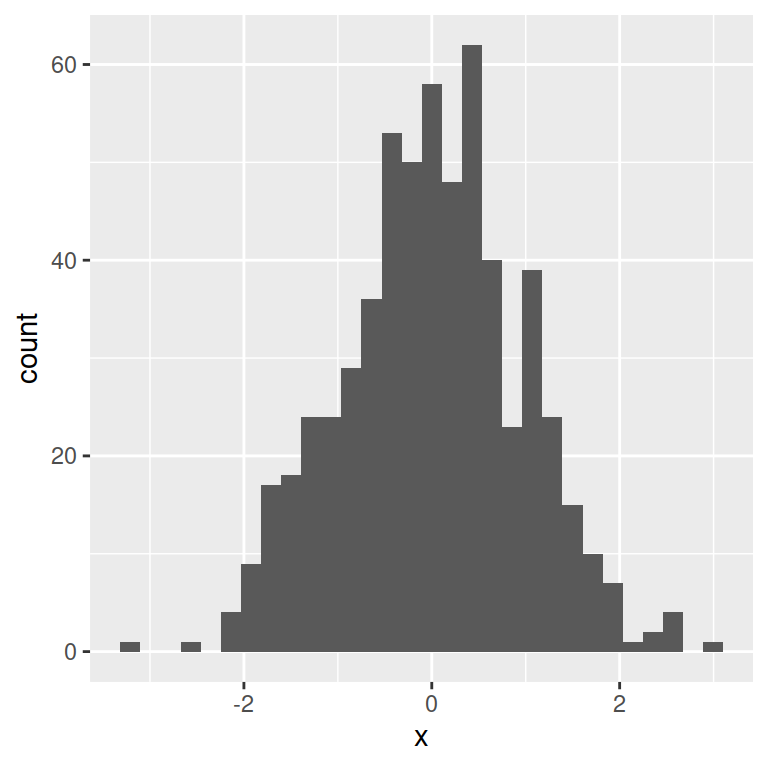This is the reason why you get the following message every time you create a default histogram in ggplot2:

`stat_bin()` using `bins = 30`. Pick better value with `binwidth`.

Possible options to deal with this is setting the number of bins with `bins` argument or modifying the width of each bin with `binwidth` argument.

## `bins` argument

The number of bins or bars of the histogram can be customized with the `bins` argument of the `geom_histogram` function. In this example 15 bins seem to be a good choice while 50 are too many.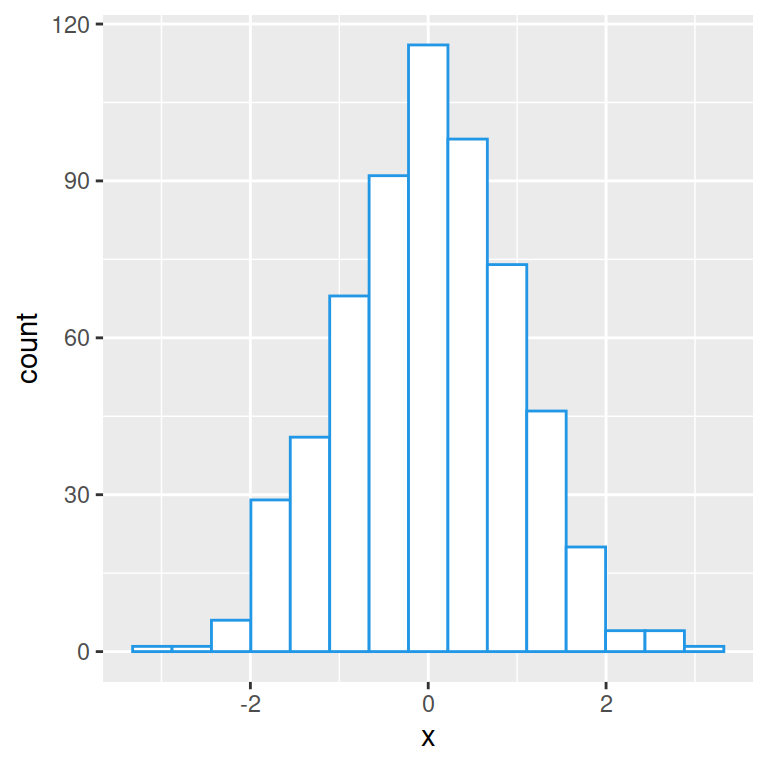15 bins

``````# install.packages("ggplot2")
library(ggplot2)

# Data
set.seed(05022021)
x <- rnorm(600)
df <- data.frame(x)

# Histogram bins
ggplot(df, aes(x = x)) +
geom_histogram(colour = 4, fill = "white",
bins = 15)``````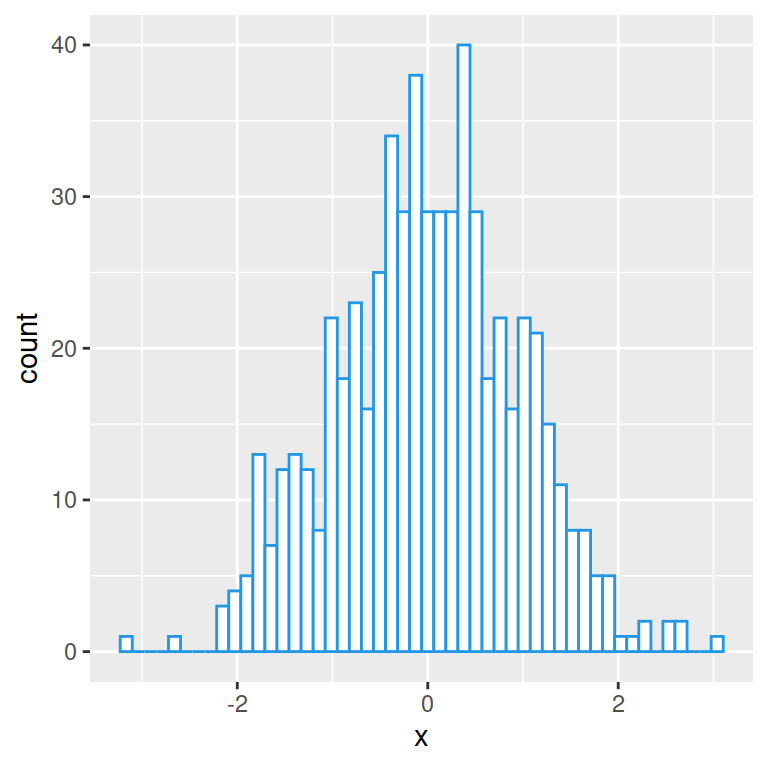50 bins

``````# install.packages("ggplot2")
library(ggplot2)

# Data
set.seed(05022021)
x <- rnorm(600)
df <- data.frame(x)

# Histogram bins
ggplot(df, aes(x = x)) +
geom_histogram(colour = 4, fill = "white",
bins = 50)``````

## `binwidth` argument

The other option is using the `binwidth` argument of the `geom_histogram` function. This argument controls the width of each bin along the X-axis. Note that this argument overrides the `bin` argument.

Binwidth of 0.5

``````# install.packages("ggplot2")
library(ggplot2)

# Data
set.seed(05022021)
x <- rnorm(600)
df <- data.frame(x)

# Histogram bin width
ggplot(df, aes(x = x)) +
geom_histogram(colour = 4, fill = "white",
binwidth = 0.5)``````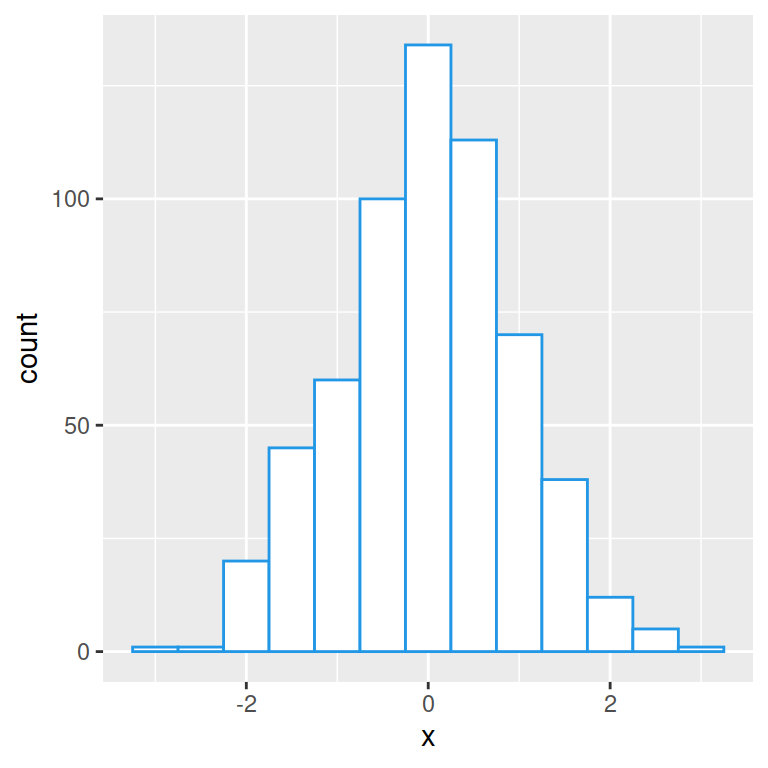Binwidth of 0.15

``````# install.packages("ggplot2")
library(ggplot2)

# Data
set.seed(05022021)
x <- rnorm(600)
df <- data.frame(x)

# Histogram bin width
ggplot(df, aes(x = x)) +
geom_histogram(colour = 4, fill = "white",
binwidth = 0.15)``````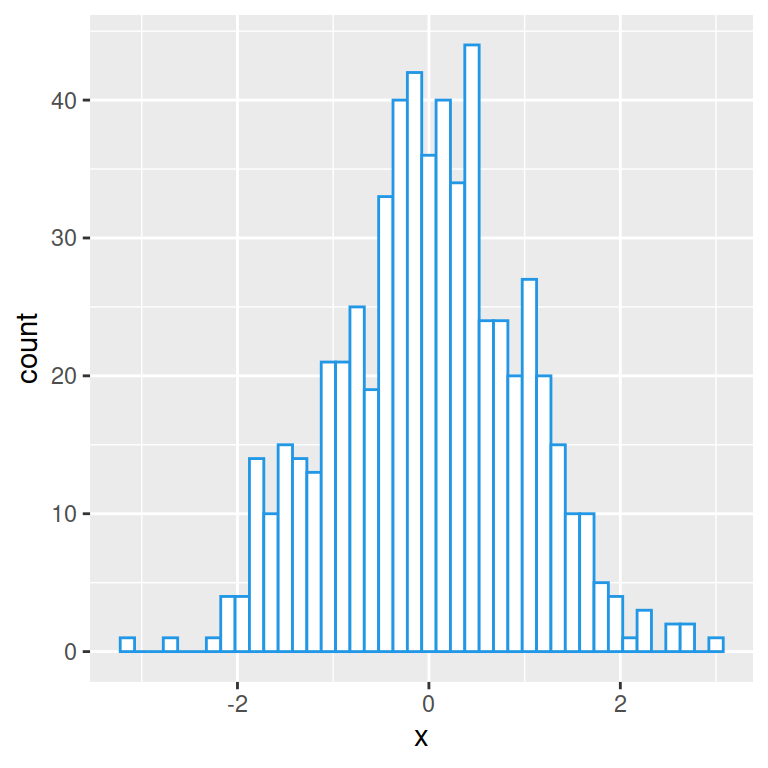Base R `hist` function uses the Sturges method to calculate the number of bins, which is a good default.